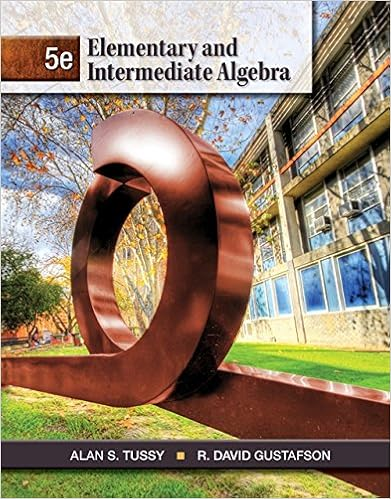# Exam B on Collge Algebra - Test Bank Exercises in CHAPTER 2...

• Test Prep
• Ramala
• 9

This preview shows page 1 - 4 out of 9 pages.

##### We have textbook solutions for you!
The document you are viewing contains questions related to this textbook.The document you are viewing contains questions related to this textbook.
Chapter 11 / Exercise 46
Elementary and Intermediate Algebra
Tussy/GustafsonExpert Verified
41Exercise Set 2.11.Solve for xthe equation 3(2x– 1) –2(x+4) =6.(a) (b) x=6(c) (d) None of the above.
2.Solve for xthe equation 2(1 – 2x) – 4(x+3) =5(1 –x).
3.Solve for xthe equation(a) (b) (c) (d) None of the above.4.Solve for xthe equation(a) (b) (c) (d) None of the above.5.Solve for xthe equation(a) (b) (c) (d) None of the above.6.Solve for xthe equation(a) (b) x=0(c) x=1(d) None of the above.x1513512x4912x.x59x95x21113x157x1.x1713x513x11123x512x23.x92x2213x14x57x638.x56x2340
Test Bank Exercises in CHAPTER2
##### We have textbook solutions for you!
The document you are viewing contains questions related to this textbook.The document you are viewing contains questions related to this textbook.
Chapter 11 / Exercise 46
Elementary and Intermediate Algebra
Tussy/GustafsonExpert Verified
7.Solve for the xthe equation(a) (b) (c) (d) None of the above. 8.Solve for xthe equation(a) (b) x=1.87(c) (d) None of the above.9.Solve for xthe equation(a) (b) (c) (d) None of the above.10. Solve for xthe equation(a) (b) (c) (d) None of the above.11. Solve for xthe equation24.
12. Solve for xthe equationx21.(a) (b) x=4(c) (d) None of the above.
13. Solve for xthe equation3.
14. Solve for xthe equationx112x1.x23xx21
15. Solve for xthe equation(x1) (x3)x1.
x742x23xx21
2x112x1.
x3.
4x21.x37x32453x24x21x24.x16479x16479x23402x75x4412x3.x2559x6126x14x13x152x32.x2138x1142x53x72x33x.x5611x5611x1744x23x7x83x.42CHAPTER2Test Bank Exercises
16. Solve for xthe equation(a) (b) x=5(c) x=1(d) None of the above.
17. Sole for xthe equation(a) (b) (c) (d) None of the above.18. Solve for xthe equation(a) (b) (c) (d) None of the above.19. Solve for xthe equation
20. Solve for xthe equation
Exercise Set 2.21.Sam is 5 years older than his brother. Thirty years from now the sum of their ages will be 75. Findthe current ages of the brother.2.Bob is presently 6 years older than his sister Kathy. Three years ago, Bob was twice as old as Kathy.How old is each now?
5.A 15-foot-long beam is cut into two pieces so that one piece is 7 feet longer than the other. Howlong will each piece be?
•••